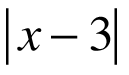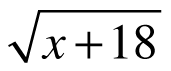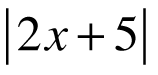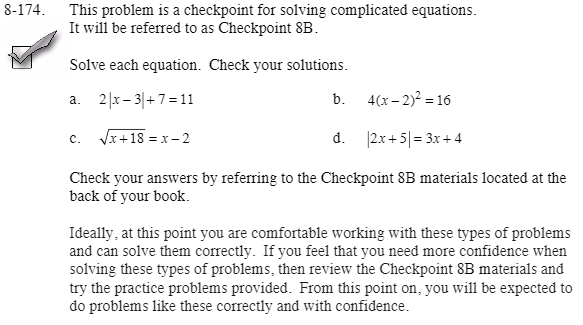Home > CCA2 > Chapter 8 > Lesson 8.3.3 > Problem8-174

8-174.
1.This problem is a checkpoint for solving complicated equations. It will be referred to as Checkpoint 8B. Homework Help ✎

2. Solve each equation. Check your solutions.

1. 2+ 7 = 11

2. 4(x − 2)2 = 16

3.= x − 2

4.= 3x + 4

3. Check your answers by referring to the Checkpoint 8B materials.

4. Ideally, at this point you are comfortable working with these types of problems and can solve them correctly. If you feel that you need more confidence when solving these types of problems, then review the Checkpoint 8B materials and try the practice problems provided. From this point on, you will be expected to do problems like these correctly and with confidence.Answers and extra practice for the Checkpoint problems are located in the back of your printed textbook or in the Reference Tab of your eBook. If you have an eBook for CCA2, login and then click the following link: Checkpoint 8B: Solving Complicated Equations